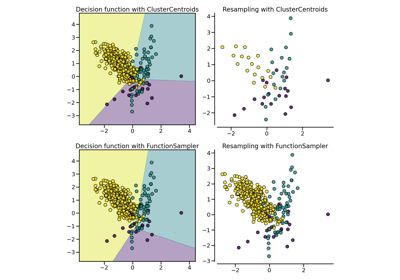# CondensedNearestNeighbour#

class imblearn.under_sampling.CondensedNearestNeighbour(*, sampling_strategy='auto', random_state=None, n_neighbors=None, n_seeds_S=1, n_jobs=None)[source]#

Undersample based on the condensed nearest neighbour method.

Read more in the User Guide.

Parameters
sampling_strategystr, list or callable

Sampling information to sample the data set.

• When `str`, specify the class targeted by the resampling. Note the the number of samples will not be equal in each. Possible choices are:

`'majority'`: resample only the majority class;

`'not minority'`: resample all classes but the minority class;

`'not majority'`: resample all classes but the majority class;

`'all'`: resample all classes;

`'auto'`: equivalent to `'not minority'`.

• When `list`, the list contains the classes targeted by the resampling.

• When callable, function taking `y` and returns a `dict`. The keys correspond to the targeted classes. The values correspond to the desired number of samples for each class.

random_stateint, RandomState instance, default=None

Control the randomization of the algorithm.

• If int, `random_state` is the seed used by the random number generator;

• If `RandomState` instance, random_state is the random number generator;

• If `None`, the random number generator is the `RandomState` instance used by `np.random`.

n_neighborsint or estimator object, default=None

If `int`, size of the neighbourhood to consider to compute the nearest neighbors. If object, an estimator that inherits from `KNeighborsMixin` that will be used to find the nearest-neighbors. If `None`, a `KNeighborsClassifier` with a 1-NN rules will be used.

n_seeds_Sint, default=1

Number of samples to extract in order to build the set S.

n_jobsint, default=None

Number of CPU cores used during the cross-validation loop. `None` means 1 unless in a `joblib.parallel_backend` context. `-1` means using all processors. See Glossary for more details.

Attributes
sampling_strategy_dict

Dictionary containing the information to sample the dataset. The keys corresponds to the class labels from which to sample and the values are the number of samples to sample.

estimator_estimator object

The validated K-nearest neighbor estimator created from `n_neighbors` parameter.

sample_indices_ndarray of shape (n_new_samples,)

Indices of the samples selected.

New in version 0.4.

n_features_in_int

Number of features in the input dataset.

New in version 0.9.

feature_names_in_ndarray of shape (`n_features_in_`,)

Names of features seen during `fit`. Defined only when `X` has feature names that are all strings.

New in version 0.10.

`EditedNearestNeighbours`

Undersample by editing samples.

`RepeatedEditedNearestNeighbours`

Undersample by repeating ENN algorithm.

`AllKNN`

Undersample using ENN and various number of neighbours.

Notes

The method is based on .

Supports multi-class resampling. A one-vs.-rest scheme is used when sampling a class as proposed in .

References

1(1,2)

P. Hart, “The condensed nearest neighbor rule,” In Information Theory, IEEE Transactions on, vol. 14(3), pp. 515-516, 1968.

Examples

```>>> from collections import Counter
>>> from sklearn.datasets import fetch_mldata
>>> from imblearn.under_sampling import CondensedNearestNeighbour
>>> pima = fetch_mldata('diabetes_scale')
>>> X, y = pima['data'], pima['target']
>>> print('Original dataset shape %s' % Counter(y))
Original dataset shape Counter({1: 500, -1: 268})
>>> cnn = CondensedNearestNeighbour(random_state=42)
>>> X_res, y_res = cnn.fit_resample(X, y)
>>> print('Resampled dataset shape %s' % Counter(y_res))
Resampled dataset shape Counter({-1: 268, 1: 227})
```

Methods

 `fit`(X, y) Check inputs and statistics of the sampler. `fit_resample`(X, y) Resample the dataset. `get_feature_names_out`([input_features]) Get output feature names for transformation. `get_params`([deep]) Get parameters for this estimator. `set_params`(**params) Set the parameters of this estimator.
fit(X, y)[source]#

Check inputs and statistics of the sampler.

You should use `fit_resample` in all cases.

Parameters
X{array-like, dataframe, sparse matrix} of shape (n_samples, n_features)

Data array.

yarray-like of shape (n_samples,)

Target array.

Returns
selfobject

Return the instance itself.

fit_resample(X, y)[source]#

Resample the dataset.

Parameters
X{array-like, dataframe, sparse matrix} of shape (n_samples, n_features)

Matrix containing the data which have to be sampled.

yarray-like of shape (n_samples,)

Corresponding label for each sample in X.

Returns
X_resampled{array-like, dataframe, sparse matrix} of shape (n_samples_new, n_features)

The array containing the resampled data.

y_resampledarray-like of shape (n_samples_new,)

The corresponding label of `X_resampled`.

get_feature_names_out(input_features=None)[source]#

Get output feature names for transformation.

Parameters
input_featuresarray-like of str or None, default=None

Input features.

• If `input_features` is `None`, then `feature_names_in_` is used as feature names in. If `feature_names_in_` is not defined, then the following input feature names are generated: `["x0", "x1", ..., "x(n_features_in_ - 1)"]`.

• If `input_features` is an array-like, then `input_features` must match `feature_names_in_` if `feature_names_in_` is defined.

Returns
feature_names_outndarray of str objects

Same as input features.

get_params(deep=True)[source]#

Get parameters for this estimator.

Parameters
deepbool, default=True

If True, will return the parameters for this estimator and contained subobjects that are estimators.

Returns
paramsdict

Parameter names mapped to their values.

set_params(**params)[source]#

Set the parameters of this estimator.

The method works on simple estimators as well as on nested objects (such as `Pipeline`). The latter have parameters of the form `<component>__<parameter>` so that it’s possible to update each component of a nested object.

Parameters
**paramsdict

Estimator parameters.

Returns
selfestimator instance

Estimator instance.

## Examples using `imblearn.under_sampling.CondensedNearestNeighbour`#Compare under-sampling samplers

Compare under-sampling samplers